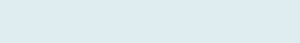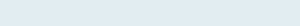# Average or Sum between two Dates or Value

After reading this article you will be able to calculate average or addition of numbers in a column between two dates or a range of numbers in Excel i.e. multiple criteria in same column.

QUERY

In this example, we have columns Payment date and Items quantity. Our task is to get an average of items quantity in a column on the basis of two dates which are equal or more than start date but less than or equals to end date.

Formula to implement:

=AVERAGEIFS(C3:C19,B3:B19,”>=”&F5,B3:B19,”<=”&F6)

Functions applicable:

The AVERAGEIFS function in excel is a plural form of AVERAGEIF. Returns an average (arithmetic mean) number based on one or multiple given criteria.The AVERAGEIFS function has the following arguments:

AVERAGE_ RANGE: The range of cells you actually want to average if it satisfies the condition.

CRITERIA_ RANGE 1, CRITERIA_ RANGE 2, ….:  1 to 127 ranges to be checked against the specified criteria. Criteria_ range1 is required, the following ones are optional.

CRITERIA 1, CRITERIA 2, ……..: 1 to 127 criteria that determine which cells to average. The criteria can be provided in the form of a number, logical expression, text value or cell reference. Criteria 1 is required, additional criteria are optional.

Formula Explanation (Step by Step)

Excel start calculating innermost parenthesis than work outward. You can also debug formula using shortcut F9 function key under formulas tab.

Let’s break the formula into multiple small steps to understand its application.

Formula

Step 1: =IFS(C3:C19,B3:B19,”>=”&F5,B3:B19,”<=”&F6)

Result:It checks both the criteria i.e. dates which are greater than or equals to date 1 or less than equals to given date 2 to know the average. Return count of TRUE incidents i.e. 17 (number dates between two dates).

Step2: =AVERAGEIFS(C3:C19,B3:B19,”>=”&F5,B3:B19,”<=”&F6)

Result: 20

(add all values from C3 to C19)= 340

(340/17)= 20

It first sums the numbers of items quantity in the given range (C3 to C19) and then divide it after date counts (17) in the column.

## Sum If between two dates

Suppose you have a list of dates to get an addition between two dates we will use the SUMIF function that adds a range of cells based on more than one criterion.

## Syntax

=SUMIFS(sum_range, criteria_range1, criteria1, [criteria_range2, criteria2], …)

Query: We have two-column of time orders written and total time. Our task is to sum total time given start and end date of Order time.

Formula to implement:

=SUMIFS(C3:C24,B3:B24,”>=”&\$F\$7,B3:B24,”<=”&\$F\$8)

Result: 10105

(622 to 260)

It will returns addition of the given range (C3:C24) based on condition if a date or a value lies between one or multiple range of date or numbers.

Note: & operator in Excel is a type of concatenate which is used to join two strings, values or results.